Sep. 30, 2010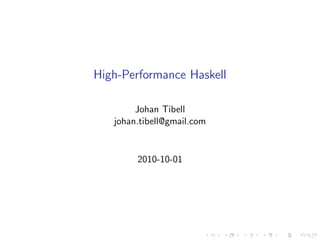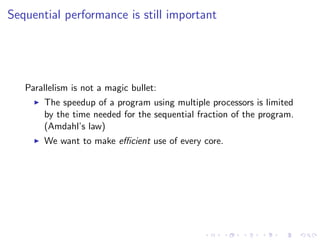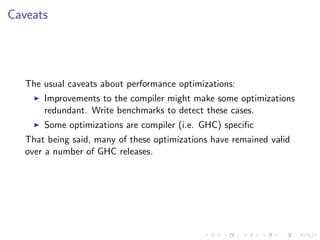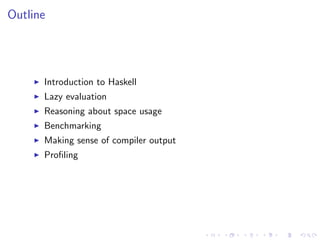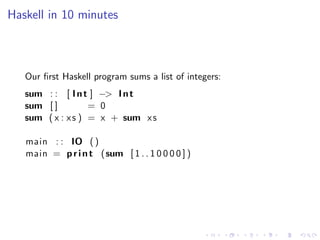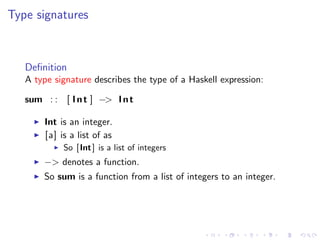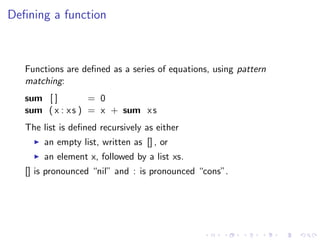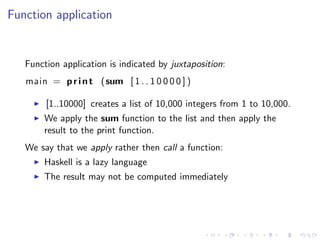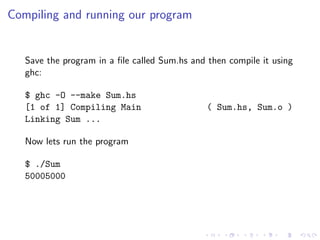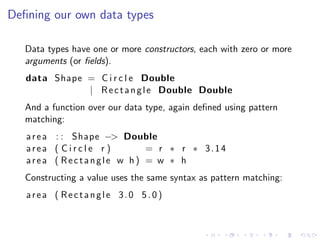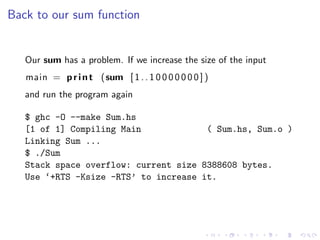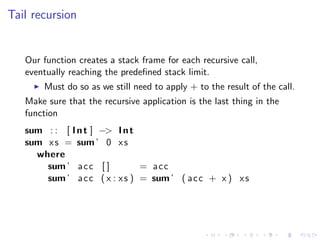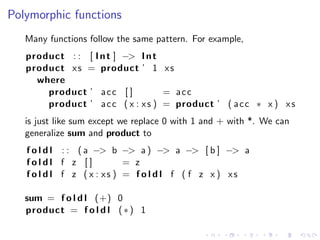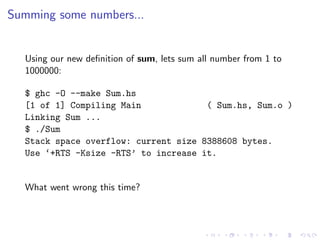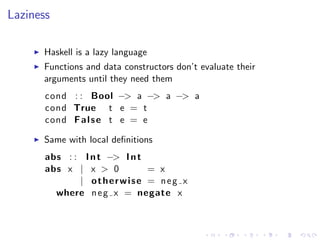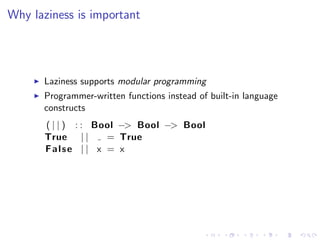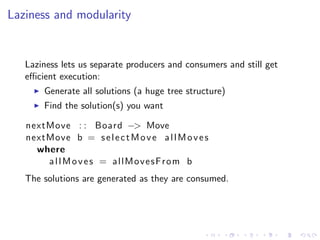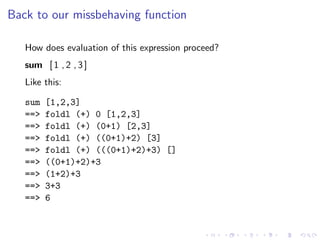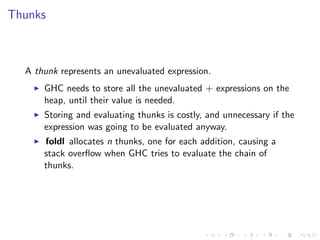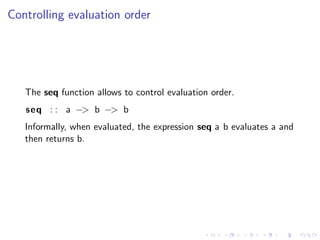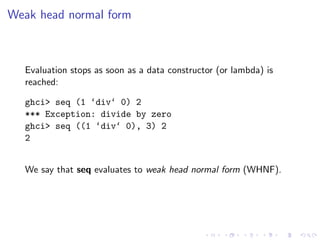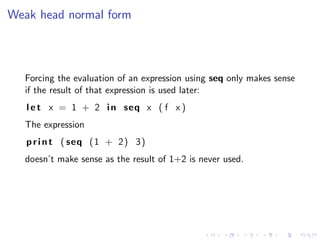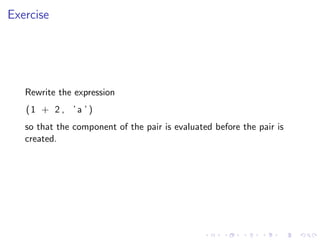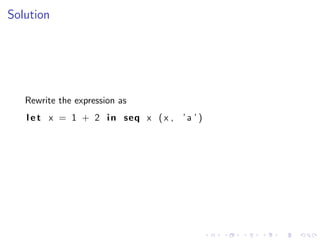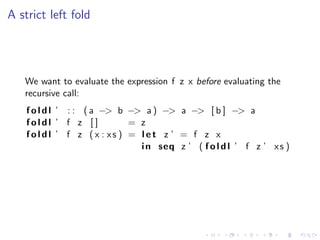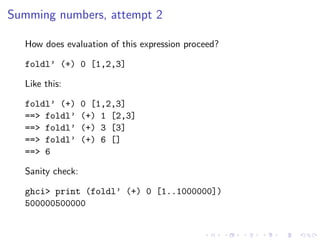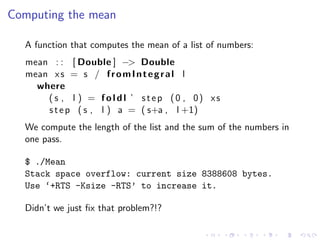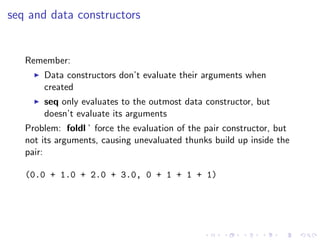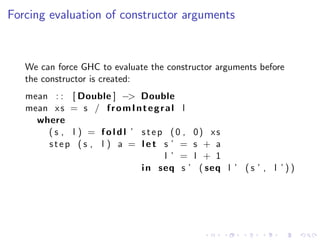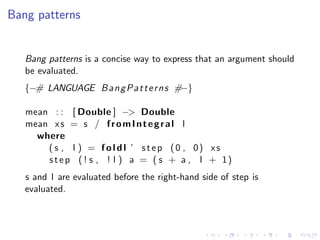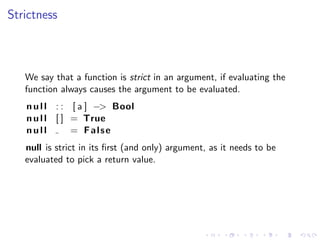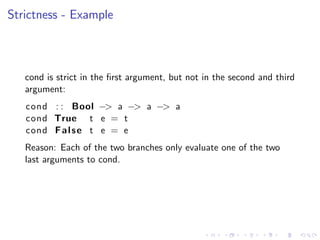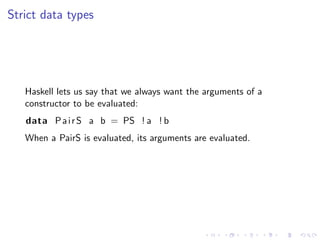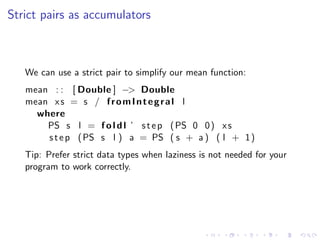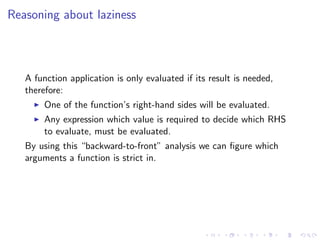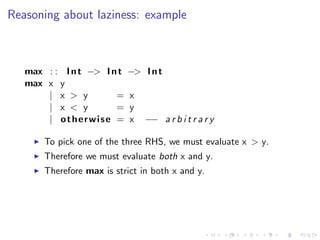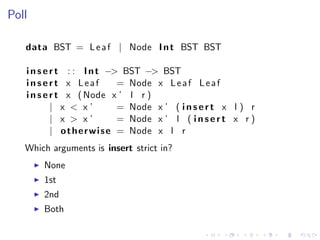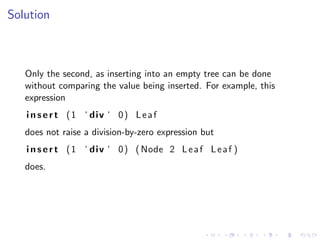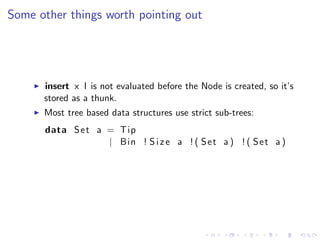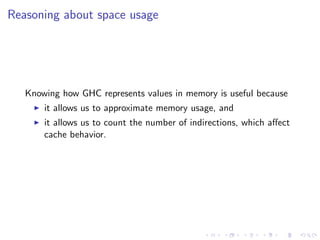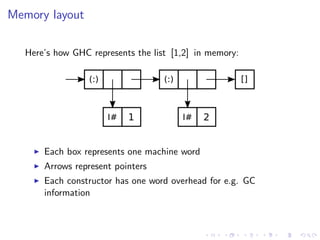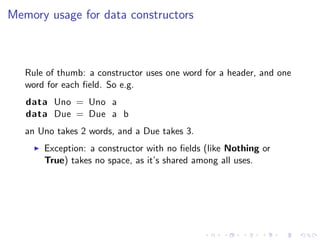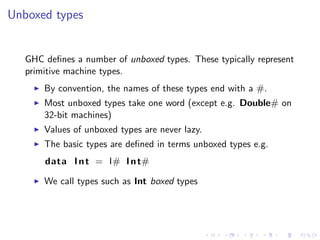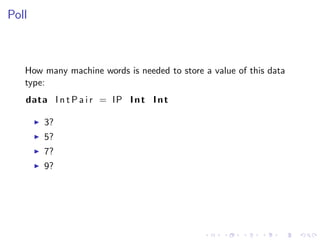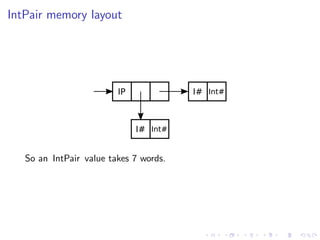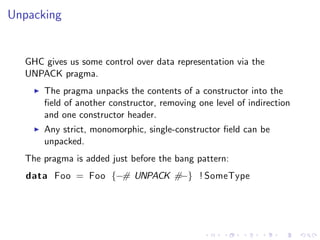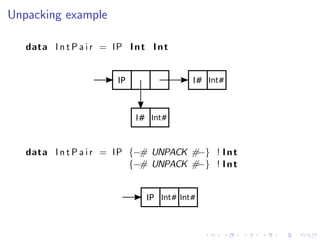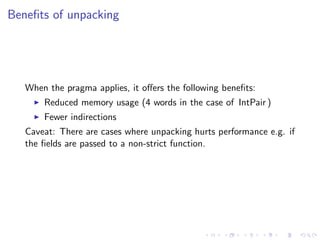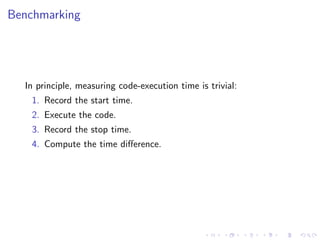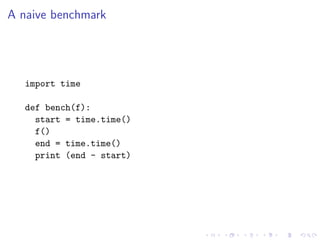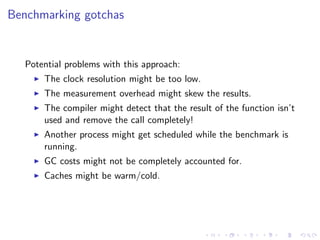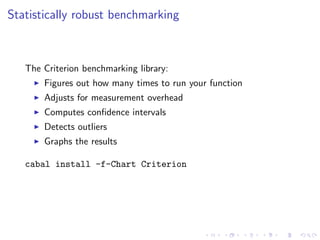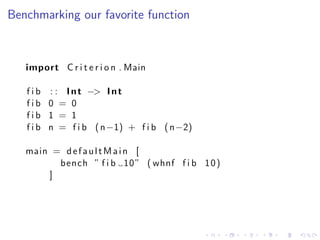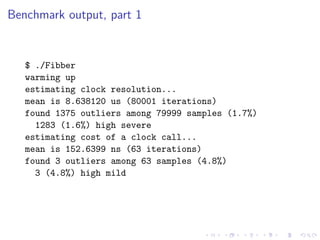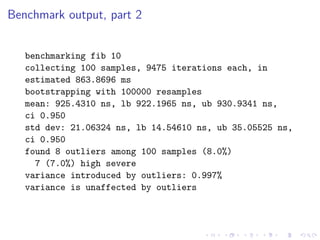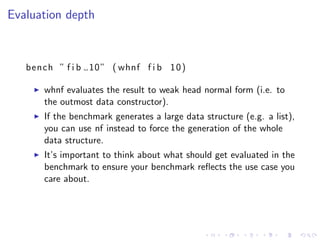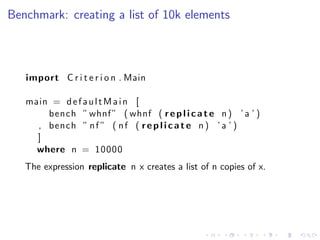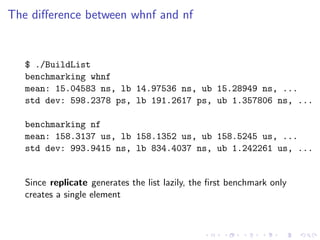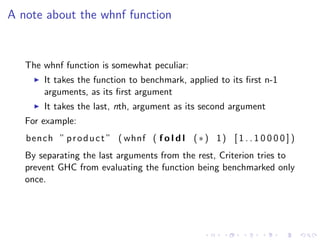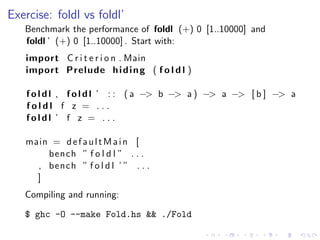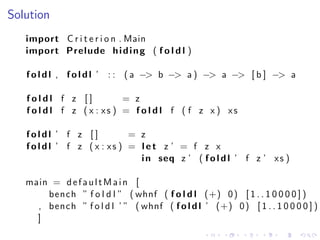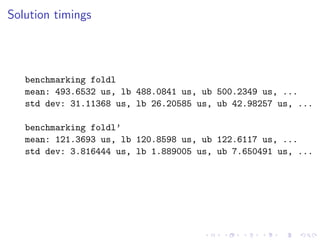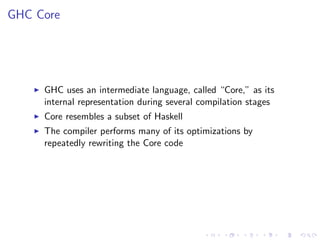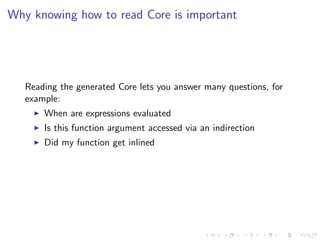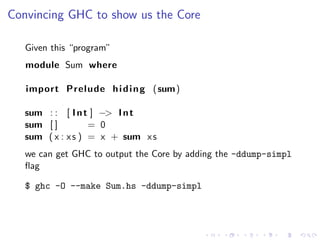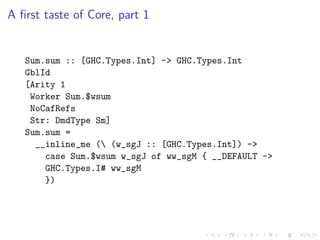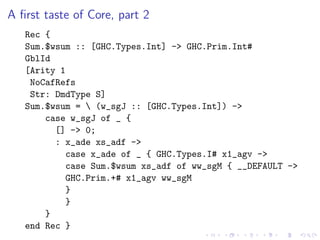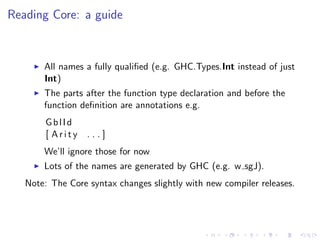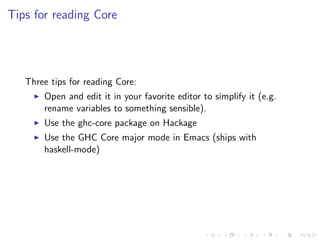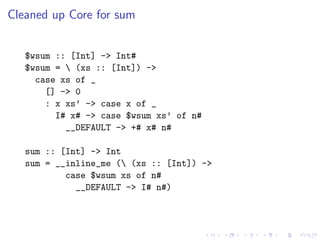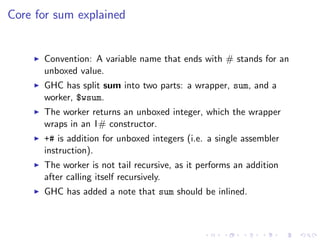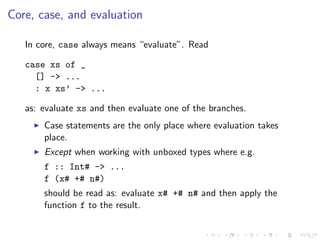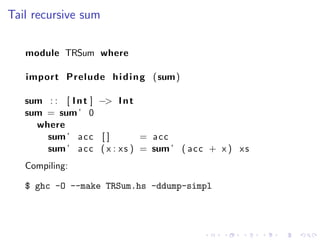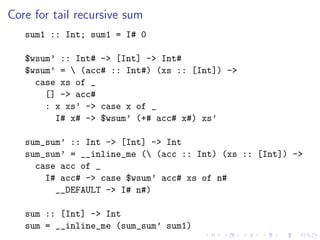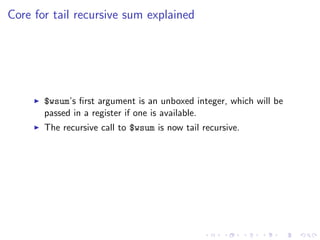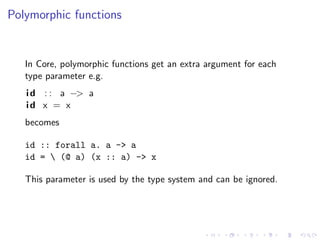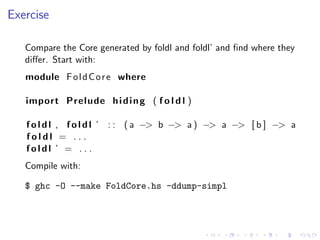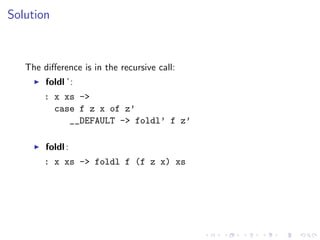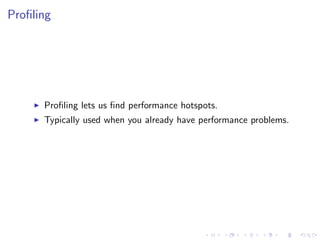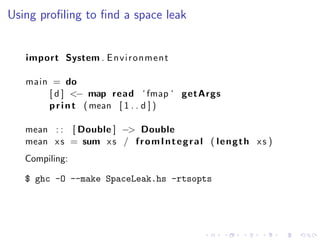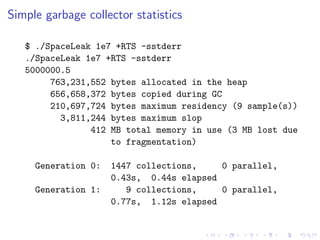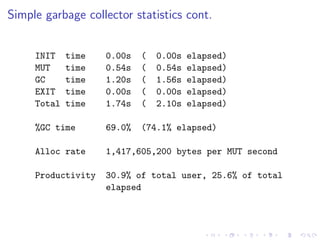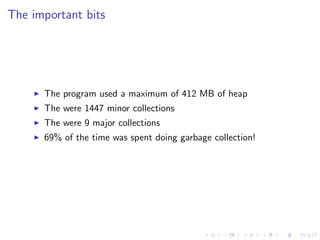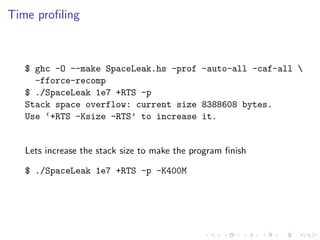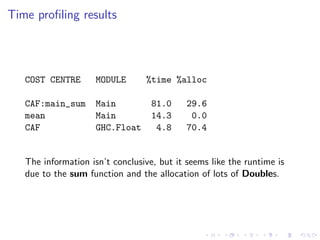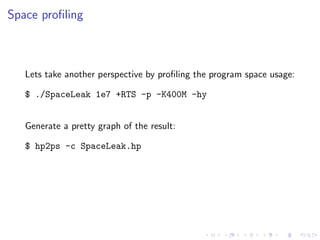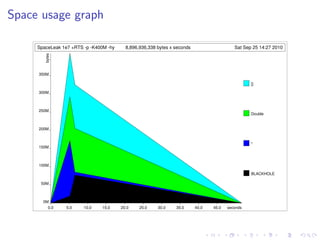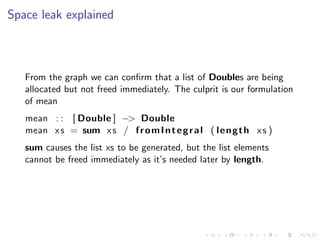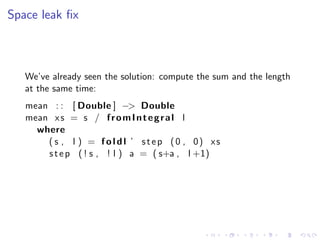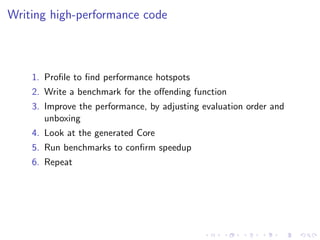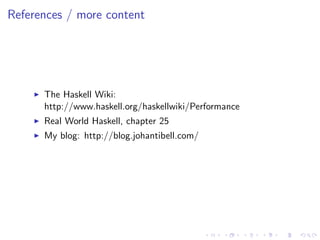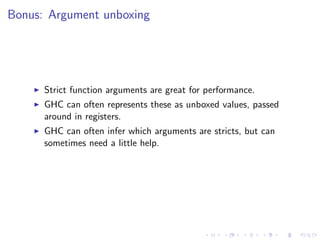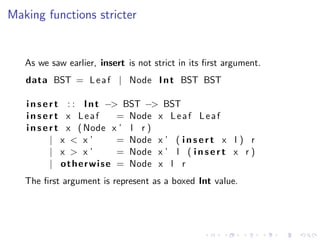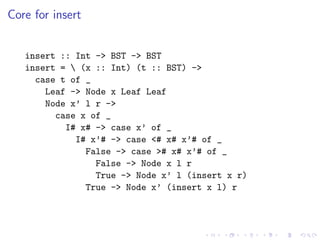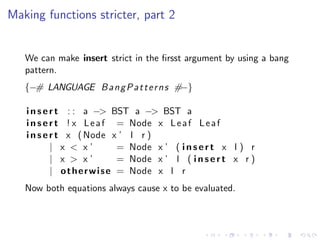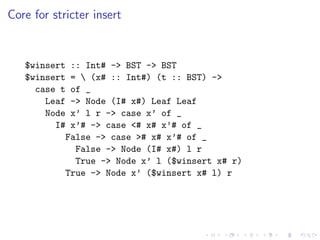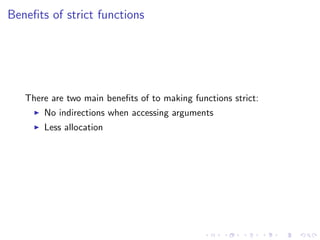1 of 99

• 1. High-Performance Haskell Johan Tibell johan.tibell@gmail.com 2010-10-01
• 3. Sequential performance is still important Parallelism is not a magic bullet: The speedup of a program using multiple processors is limited by the time needed for the sequential fraction of the program. (Amdahl’s law) We want to make eﬃcient use of every core.
• 4. Caveats The usual caveats about performance optimizations: Improvements to the compiler might make some optimizations redundant. Write benchmarks to detect these cases. Some optimizations are compiler (i.e. GHC) speciﬁc That being said, many of these optimizations have remained valid over a number of GHC releases.
• 5. Software prerequisites The Haskell Platform: Download installer for Windows, OS X, or Linux here: http://hackage.haskell.org/platform The Criterion benchmarking library: cabal install -f-Chart criterion
• 6. Outline Introduction to Haskell Lazy evaluation Reasoning about space usage Benchmarking Making sense of compiler output Proﬁling
• 7. Haskell in 10 minutes Our ﬁrst Haskell program sums a list of integers: sum : : [ I n t ] −> I n t sum [ ] = 0 sum ( x : x s ) = x + sum x s main : : IO ( ) main = p r i n t (sum [ 1 . . 1 0 0 0 0 ] )
• 8. Type signatures Deﬁnition A type signature describes the type of a Haskell expression: sum : : [ I n t ] −> I n t Int is an integer. [a] is a list of as So [ Int ] is a list of integers −> denotes a function. So sum is a function from a list of integers to an integer.
• 9. Deﬁning a function Functions are deﬁned as a series of equations, using pattern matching: sum [ ] = 0 sum ( x : x s ) = x + sum x s The list is deﬁned recursively as either an empty list, written as [] , or an element x, followed by a list xs. [] is pronounced “nil” and : is pronounced “cons”.
• 10. Function application Function application is indicated by juxtaposition: main = p r i n t (sum [ 1 . . 1 0 0 0 0 ] ) [1..10000] creates a list of 10,000 integers from 1 to 10,000. We apply the sum function to the list and then apply the result to the print function. We say that we apply rather then call a function: Haskell is a lazy language The result may not be computed immediately
• 11. Compiling and running our program Save the program in a ﬁle called Sum.hs and then compile it using ghc: \$ ghc -O --make Sum.hs [1 of 1] Compiling Main ( Sum.hs, Sum.o ) Linking Sum ... Now lets run the program \$ ./Sum 50005000
• 12. Deﬁning our own data types Data types have one or more constructors, each with zero or more arguments (or ﬁelds). data Shape = C i r c l e Double | R e c t a n g l e Double Double And a function over our data type, again deﬁned using pattern matching: a r e a : : Shape −> Double area ( C i r c l e r ) = r ∗ r ∗ 3.14 area ( Rectangle w h) = w ∗ h Constructing a value uses the same syntax as pattern matching: area ( Rectangle 3.0 5.0)
• 13. Back to our sum function Our sum has a problem. If we increase the size of the input main = p r i n t (sum [ 1 . . 1 0 0 0 0 0 0 0 ] ) and run the program again \$ ghc -O --make Sum.hs [1 of 1] Compiling Main ( Sum.hs, Sum.o ) Linking Sum ... \$ ./Sum Stack space overflow: current size 8388608 bytes. Use ‘+RTS -Ksize -RTS’ to increase it.
• 14. Tail recursion Our function creates a stack frame for each recursive call, eventually reaching the predeﬁned stack limit. Must do so as we still need to apply + to the result of the call. Make sure that the recursive application is the last thing in the function sum : : [ I n t ] −> I n t sum x s = sum ’ 0 x s where sum ’ a c c [ ] = acc sum ’ a c c ( x : x s ) = sum ’ ( a c c + x ) x s
• 15. Polymorphic functions Many functions follow the same pattern. For example, product : : [ I n t ] −> I n t product x s = product ’ 1 x s where product ’ a c c [ ] = acc product ’ a c c ( x : x s ) = product ’ ( a c c ∗ x ) x s is just like sum except we replace 0 with 1 and + with *. We can generalize sum and product to f o l d l : : ( a −> b −> a ) −> a −> [ b ] −> a foldl f z [] = z f o l d l f z ( x : xs ) = f o l d l f ( f z x ) xs sum = f o l d l (+) 0 product = f o l d l ( ∗ ) 1
• 16. Summing some numbers... Using our new deﬁnition of sum, lets sum all number from 1 to 1000000: \$ ghc -O --make Sum.hs [1 of 1] Compiling Main ( Sum.hs, Sum.o ) Linking Sum ... \$ ./Sum Stack space overflow: current size 8388608 bytes. Use ‘+RTS -Ksize -RTS’ to increase it. What went wrong this time?
• 17. Laziness Haskell is a lazy language Functions and data constructors don’t evaluate their arguments until they need them cond : : Bool −> a −> a −> a cond True t e = t cond F a l s e t e = e Same with local deﬁnitions abs : : I n t −> I n t abs x | x > 0 = x | otherwise = neg x where n e g x = negate x
• 18. Why laziness is important Laziness supports modular programming Programmer-written functions instead of built-in language constructs ( | | ) : : Bool −> Bool −> Bool True | | = True False | | x = x
• 19. Laziness and modularity Laziness lets us separate producers and consumers and still get eﬃcient execution: Generate all solutions (a huge tree structure) Find the solution(s) you want nextMove : : Board −> Move nextMove b = s e l e c t M o v e a l l M o v e s where allMoves = allMovesFrom b The solutions are generated as they are consumed.
• 20. Back to our missbehaving function How does evaluation of this expression proceed? sum [ 1 , 2 , 3 ] Like this: sum [1,2,3] ==> foldl (+) 0 [1,2,3] ==> foldl (+) (0+1) [2,3] ==> foldl (+) ((0+1)+2)  ==> foldl (+) (((0+1)+2)+3) [] ==> ((0+1)+2)+3 ==> (1+2)+3 ==> 3+3 ==> 6
• 21. Thunks A thunk represents an unevaluated expression. GHC needs to store all the unevaluated + expressions on the heap, until their value is needed. Storing and evaluating thunks is costly, and unnecessary if the expression was going to be evaluated anyway. foldl allocates n thunks, one for each addition, causing a stack overﬂow when GHC tries to evaluate the chain of thunks.
• 22. Controlling evaluation order The seq function allows to control evaluation order. seq : : a −> b −> b Informally, when evaluated, the expression seq a b evaluates a and then returns b.
• 23. Weak head normal form Evaluation stops as soon as a data constructor (or lambda) is reached: ghci> seq (1 ‘div‘ 0) 2 *** Exception: divide by zero ghci> seq ((1 ‘div‘ 0), 3) 2 2 We say that seq evaluates to weak head normal form (WHNF).
• 24. Weak head normal form Forcing the evaluation of an expression using seq only makes sense if the result of that expression is used later: l e t x = 1 + 2 i n seq x ( f x ) The expression p r i n t ( seq ( 1 + 2 ) 3 ) doesn’t make sense as the result of 1+2 is never used.
• 25. Exercise Rewrite the expression (1 + 2 , ’ a ’ ) so that the component of the pair is evaluated before the pair is created.
• 26. Solution Rewrite the expression as l e t x = 1 + 2 i n seq x ( x , ’ a ’ )
• 27. A strict left fold We want to evaluate the expression f z x before evaluating the recursive call: f o l d l ’ : : ( a −> b −> a ) −> a −> [ b ] −> a foldl ’ f z [ ] = z foldl ’ f z ( x : xs ) = l e t z ’ = f z x i n seq z ’ ( f o l d l ’ f z ’ x s )
• 28. Summing numbers, attempt 2 How does evaluation of this expression proceed? foldl’ (+) 0 [1,2,3] Like this: foldl’ (+) 0 [1,2,3] ==> foldl’ (+) 1 [2,3] ==> foldl’ (+) 3  ==> foldl’ (+) 6 [] ==> 6 Sanity check: ghci> print (foldl’ (+) 0 [1..1000000]) 500000500000
• 29. Computing the mean A function that computes the mean of a list of numbers: mean : : [ Double ] −> Double mean x s = s / f r o m I n t e g r a l l where ( s , l ) = f o l d l ’ s t e p (0 , 0) xs s t e p ( s , l ) a = ( s+a , l +1) We compute the length of the list and the sum of the numbers in one pass. \$ ./Mean Stack space overflow: current size 8388608 bytes. Use ‘+RTS -Ksize -RTS’ to increase it. Didn’t we just ﬁx that problem?!?
• 30. seq and data constructors Remember: Data constructors don’t evaluate their arguments when created seq only evaluates to the outmost data constructor, but doesn’t evaluate its arguments Problem: foldl ’ force the evaluation of the pair constructor, but not its arguments, causing unevaluated thunks build up inside the pair: (0.0 + 1.0 + 2.0 + 3.0, 0 + 1 + 1 + 1)
• 31. Forcing evaluation of constructor arguments We can force GHC to evaluate the constructor arguments before the constructor is created: mean : : [ Double ] −> Double mean x s = s / f r o m I n t e g r a l l where ( s , l ) = f o l d l ’ s t e p (0 , 0) xs step (s , l ) a = let s ’ = s + a l ’ = l + 1 i n seq s ’ ( seq l ’ ( s ’ , l ’ ) )
• 32. Bang patterns Bang patterns is a concise way to express that an argument should be evaluated. {−# LANGUAGE B a n g P a t t e r n s #−} mean : : [ Double ] −> Double mean x s = s / f r o m I n t e g r a l l where ( s , l ) = f o l d l ’ s t e p (0 , 0) xs s t e p ( ! s , ! l ) a = ( s + a , l + 1) s and l are evaluated before the right-hand side of step is evaluated.
• 33. Strictness We say that a function is strict in an argument, if evaluating the function always causes the argument to be evaluated. n u l l : : [ a ] −> Bool n u l l [ ] = True null = False null is strict in its ﬁrst (and only) argument, as it needs to be evaluated to pick a return value.
• 34. Strictness - Example cond is strict in the ﬁrst argument, but not in the second and third argument: cond : : Bool −> a −> a −> a cond True t e = t cond F a l s e t e = e Reason: Each of the two branches only evaluate one of the two last arguments to cond.
• 35. Strict data types Haskell lets us say that we always want the arguments of a constructor to be evaluated: data P a i r S a b = PS ! a ! b When a PairS is evaluated, its arguments are evaluated.
• 36. Strict pairs as accumulators We can use a strict pair to simplify our mean function: mean : : [ Double ] −> Double mean x s = s / f r o m I n t e g r a l l where PS s l = f o l d l ’ s t e p ( PS 0 0 ) x s s t e p ( PS s l ) a = PS ( s + a ) ( l + 1 ) Tip: Prefer strict data types when laziness is not needed for your program to work correctly.
• 37. Reasoning about laziness A function application is only evaluated if its result is needed, therefore: One of the function’s right-hand sides will be evaluated. Any expression which value is required to decide which RHS to evaluate, must be evaluated. By using this “backward-to-front” analysis we can ﬁgure which arguments a function is strict in.
• 38. Reasoning about laziness: example max : : I n t −> I n t −> I n t max x y | x > y = x | x < y = y | otherwise = x −− a r b i t r a r y To pick one of the three RHS, we must evaluate x > y. Therefore we must evaluate both x and y. Therefore max is strict in both x and y.
• 39. Poll data BST = L e a f | Node I n t BST BST i n s e r t : : I n t −> BST −> BST insert x Leaf = Node x L e a f L e a f i n s e r t x ( Node x ’ l r ) | x < x’ = Node x ’ ( i n s e r t x l ) r | x > x’ = Node x ’ l ( i n s e r t x r ) | o t h e r w i s e = Node x l r Which arguments is insert strict in? None 1st 2nd Both
• 40. Solution Only the second, as inserting into an empty tree can be done without comparing the value being inserted. For example, this expression i n s e r t ( 1 ‘ div ‘ 0 ) L e a f does not raise a division-by-zero expression but i n s e r t ( 1 ‘ div ‘ 0 ) ( Node 2 L e a f L e a f ) does.
• 41. Some other things worth pointing out insert x l is not evaluated before the Node is created, so it’s stored as a thunk. Most tree based data structures use strict sub-trees: data S e t a = Tip | Bin ! S i z e a ! ( S e t a ) ! ( S e t a )
• 42. Reasoning about space usage Knowing how GHC represents values in memory is useful because it allows us to approximate memory usage, and it allows us to count the number of indirections, which aﬀect cache behavior.
• 43. Memory layout Here’s how GHC represents the list [1,2] in memory: (:) (:) [] I# 1 I# 2 Each box represents one machine word Arrows represent pointers Each constructor has one word overhead for e.g. GC information
• 44. Memory usage for data constructors Rule of thumb: a constructor uses one word for a header, and one word for each ﬁeld. So e.g. data Uno = Uno a data Due = Due a b an Uno takes 2 words, and a Due takes 3. Exception: a constructor with no ﬁelds (like Nothing or True) takes no space, as it’s shared among all uses.
• 45. Unboxed types GHC deﬁnes a number of unboxed types. These typically represent primitive machine types. By convention, the names of these types end with a #. Most unboxed types take one word (except e.g. Double# on 32-bit machines) Values of unboxed types are never lazy. The basic types are deﬁned in terms unboxed types e.g. data I n t = I# I n t# We call types such as Int boxed types
• 46. Poll How many machine words is needed to store a value of this data type: data I n t P a i r = IP I n t I n t 3? 5? 7? 9?
• 47. IntPair memory layout IP I# Int# I# Int# So an IntPair value takes 7 words.
• 48. Unpacking GHC gives us some control over data representation via the UNPACK pragma. The pragma unpacks the contents of a constructor into the ﬁeld of another constructor, removing one level of indirection and one constructor header. Any strict, monomorphic, single-constructor ﬁeld can be unpacked. The pragma is added just before the bang pattern: data Foo = Foo {−# UNPACK #−} ! SomeType
• 49. Unpacking example data I n t P a i r = IP I n t I n t IP I# Int# I# Int# data I n t P a i r = IP {−# UNPACK #−} ! I n t {−# UNPACK #−} ! I n t IP Int# Int#
• 50. Beneﬁts of unpacking When the pragma applies, it oﬀers the following beneﬁts: Reduced memory usage (4 words in the case of IntPair ) Fewer indirections Caveat: There are cases where unpacking hurts performance e.g. if the ﬁelds are passed to a non-strict function.
• 51. Benchmarking In principle, measuring code-execution time is trivial: 1. Record the start time. 2. Execute the code. 3. Record the stop time. 4. Compute the time diﬀerence.
• 52. A naive benchmark import time def bench(f): start = time.time() f() end = time.time() print (end - start)
• 53. Benchmarking gotchas Potential problems with this approach: The clock resolution might be too low. The measurement overhead might skew the results. The compiler might detect that the result of the function isn’t used and remove the call completely! Another process might get scheduled while the benchmark is running. GC costs might not be completely accounted for. Caches might be warm/cold.
• 54. Statistically robust benchmarking The Criterion benchmarking library: Figures out how many times to run your function Adjusts for measurement overhead Computes conﬁdence intervals Detects outliers Graphs the results cabal install -f-Chart Criterion
• 55. Benchmarking our favorite function import C r i t e r i o n . Main f i b : : I n t −> I n t fib 0 = 0 fib 1 = 1 f i b n = f i b ( n−1) + f i b ( n−2) main = d e f a u l t M a i n [ bench ” f i b 10 ” ( whnf f i b 1 0 ) ]
• 56. Benchmark output, part 1 \$ ./Fibber warming up estimating clock resolution... mean is 8.638120 us (80001 iterations) found 1375 outliers among 79999 samples (1.7%) 1283 (1.6%) high severe estimating cost of a clock call... mean is 152.6399 ns (63 iterations) found 3 outliers among 63 samples (4.8%) 3 (4.8%) high mild
• 57. Benchmark output, part 2 benchmarking fib 10 collecting 100 samples, 9475 iterations each, in estimated 863.8696 ms bootstrapping with 100000 resamples mean: 925.4310 ns, lb 922.1965 ns, ub 930.9341 ns, ci 0.950 std dev: 21.06324 ns, lb 14.54610 ns, ub 35.05525 ns, ci 0.950 found 8 outliers among 100 samples (8.0%) 7 (7.0%) high severe variance introduced by outliers: 0.997% variance is unaffected by outliers
• 58. Evaluation depth bench ” f i b 10 ” ( whnf f i b 1 0 ) whnf evaluates the result to weak head normal form (i.e. to the outmost data constructor). If the benchmark generates a large data structure (e.g. a list), you can use nf instead to force the generation of the whole data structure. It’s important to think about what should get evaluated in the benchmark to ensure your benchmark reﬂects the use case you care about.
• 59. Benchmark: creating a list of 10k elements import C r i t e r i o n . Main main = d e f a u l t M a i n [ bench ” whnf ” ( whnf ( r e p l i c a t e n ) ’ a ’ ) , bench ” n f ” ( n f ( r e p l i c a t e n ) ’ a ’ ) ] where n = 10000 The expression replicate n x creates a list of n copies of x.
• 60. The diﬀerence between whnf and nf \$ ./BuildList benchmarking whnf mean: 15.04583 ns, lb 14.97536 ns, ub 15.28949 ns, ... std dev: 598.2378 ps, lb 191.2617 ps, ub 1.357806 ns, ... benchmarking nf mean: 158.3137 us, lb 158.1352 us, ub 158.5245 us, ... std dev: 993.9415 ns, lb 834.4037 ns, ub 1.242261 us, ... Since replicate generates the list lazily, the ﬁrst benchmark only creates a single element
• 61. A note about the whnf function The whnf function is somewhat peculiar: It takes the function to benchmark, applied to its ﬁrst n-1 arguments, as its ﬁrst argument It takes the last, nth, argument as its second argument For example: bench ” p r o d u c t ” ( whnf ( f o l d l ( ∗ ) 1 ) [ 1 . . 1 0 0 0 0 ] ) By separating the last arguments from the rest, Criterion tries to prevent GHC from evaluating the function being benchmarked only once.
• 62. Exercise: foldl vs foldl’ Benchmark the performance of foldl (+) 0 [1..10000] and foldl ’ (+) 0 [1..10000] . Start with: import C r i t e r i o n . Main import Prelude h i d i n g ( f o l d l ) f o l d l , f o l d l ’ : : ( a −> b −> a ) −> a −> [ b ] −> a foldl f z = . . . foldl ’ f z = . . . main = d e f a u l t M a i n [ bench ” f o l d l ” . . . , bench ” f o l d l ’ ” . . . ] Compiling and running: \$ ghc -O --make Fold.hs && ./Fold
• 63. Solution import C r i t e r i o n . Main import Prelude h i d i n g ( f o l d l ) f o l d l , f o l d l ’ : : ( a −> b −> a ) −> a −> [ b ] −> a foldl f z [] = z f o l d l f z ( x : xs ) = f o l d l f ( f z x ) xs foldl ’ f z [ ] = z foldl ’ f z ( x : xs ) = l e t z ’ = f z x i n seq z ’ ( f o l d l ’ f z ’ x s ) main = d e f a u l t M a i n [ bench ” f o l d l ” ( whnf ( f o l d l (+) 0 ) [ 1 . . 1 0 0 0 0 ] ) , bench ” f o l d l ’ ” ( whnf ( f o l d l ’ (+) 0 ) [ 1 . . 1 0 0 0 0 ] ) ]
• 64. Solution timings benchmarking foldl mean: 493.6532 us, lb 488.0841 us, ub 500.2349 us, ... std dev: 31.11368 us, lb 26.20585 us, ub 42.98257 us, ... benchmarking foldl’ mean: 121.3693 us, lb 120.8598 us, ub 122.6117 us, ... std dev: 3.816444 us, lb 1.889005 us, ub 7.650491 us, ...
• 65. GHC Core GHC uses an intermediate language, called “Core,” as its internal representation during several compilation stages Core resembles a subset of Haskell The compiler performs many of its optimizations by repeatedly rewriting the Core code
• 66. Why knowing how to read Core is important Reading the generated Core lets you answer many questions, for example: When are expressions evaluated Is this function argument accessed via an indirection Did my function get inlined
• 67. Convincing GHC to show us the Core Given this “program” module Sum where import Prelude h i d i n g (sum) sum : : [ I n t ] −> I n t sum [ ] = 0 sum ( x : x s ) = x + sum x s we can get GHC to output the Core by adding the -ddump-simpl ﬂag \$ ghc -O --make Sum.hs -ddump-simpl
• 68. A ﬁrst taste of Core, part 1 Sum.sum :: [GHC.Types.Int] -> GHC.Types.Int GblId [Arity 1 Worker Sum.\$wsum NoCafRefs Str: DmdType Sm] Sum.sum = __inline_me ( (w_sgJ :: [GHC.Types.Int]) -> case Sum.\$wsum w_sgJ of ww_sgM { __DEFAULT -> GHC.Types.I# ww_sgM })
• 69. A ﬁrst taste of Core, part 2 Rec { Sum.\$wsum :: [GHC.Types.Int] -> GHC.Prim.Int# GblId [Arity 1 NoCafRefs Str: DmdType S] Sum.\$wsum = (w_sgJ :: [GHC.Types.Int]) -> case w_sgJ of _ { [] -> 0; : x_ade xs_adf -> case x_ade of _ { GHC.Types.I# x1_agv -> case Sum.\$wsum xs_adf of ww_sgM { __DEFAULT -> GHC.Prim.+# x1_agv ww_sgM } } } end Rec }
• 70. Reading Core: a guide All names a fully qualiﬁed (e.g. GHC.Types.Int instead of just Int) The parts after the function type declaration and before the function deﬁnition are annotations e.g. GblId [ Arity ...] We’ll ignore those for now Lots of the names are generated by GHC (e.g. w sgJ). Note: The Core syntax changes slightly with new compiler releases.
• 71. Tips for reading Core Three tips for reading Core: Open and edit it in your favorite editor to simplify it (e.g. rename variables to something sensible). Use the ghc-core package on Hackage Use the GHC Core major mode in Emacs (ships with haskell-mode)
• 72. Cleaned up Core for sum \$wsum :: [Int] -> Int# \$wsum = (xs :: [Int]) -> case xs of _ [] -> 0 : x xs’ -> case x of _ I# x# -> case \$wsum xs’ of n# __DEFAULT -> +# x# n# sum :: [Int] -> Int sum = __inline_me ( (xs :: [Int]) -> case \$wsum xs of n# __DEFAULT -> I# n#)
• 73. Core for sum explained Convention: A variable name that ends with # stands for an unboxed value. GHC has split sum into two parts: a wrapper, sum, and a worker, \$wsum. The worker returns an unboxed integer, which the wrapper wraps in an I# constructor. +# is addition for unboxed integers (i.e. a single assembler instruction). The worker is not tail recursive, as it performs an addition after calling itself recursively. GHC has added a note that sum should be inlined.
• 74. Core, case, and evaluation In core, case always means “evaluate”. Read case xs of _ [] -> ... : x xs’ -> ... as: evaluate xs and then evaluate one of the branches. Case statements are the only place where evaluation takes place. Except when working with unboxed types where e.g. f :: Int# -> ... f (x# +# n#) should be read as: evaluate x# +# n# and then apply the function f to the result.
• 75. Tail recursive sum module TRSum where import Prelude h i d i n g (sum) sum : : [ I n t ] −> I n t sum = sum ’ 0 where sum ’ a c c [ ] = acc sum ’ a c c ( x : x s ) = sum ’ ( a c c + x ) x s Compiling: \$ ghc -O --make TRSum.hs -ddump-simpl
• 76. Core for tail recursive sum sum1 :: Int; sum1 = I# 0 \$wsum’ :: Int# -> [Int] -> Int# \$wsum’ = (acc# :: Int#) (xs :: [Int]) -> case xs of _ [] -> acc# : x xs’ -> case x of _ I# x# -> \$wsum’ (+# acc# x#) xs’ sum_sum’ :: Int -> [Int] -> Int sum_sum’ = __inline_me ( (acc :: Int) (xs :: [Int]) -> case acc of _ I# acc# -> case \$wsum’ acc# xs of n# __DEFAULT -> I# n#) sum :: [Int] -> Int sum = __inline_me (sum_sum’ sum1)
• 77. Core for tail recursive sum explained \$wsum’s ﬁrst argument is an unboxed integer, which will be passed in a register if one is available. The recursive call to \$wsum is now tail recursive.
• 78. Polymorphic functions In Core, polymorphic functions get an extra argument for each type parameter e.g. i d : : a −> a id x = x becomes id :: forall a. a -> a id = (@ a) (x :: a) -> x This parameter is used by the type system and can be ignored.
• 79. Exercise Compare the Core generated by foldl and foldl’ and ﬁnd where they diﬀer. Start with: module F o l d C o r e where import Prelude h i d i n g ( f o l d l ) f o l d l , f o l d l ’ : : ( a −> b −> a ) −> a −> [ b ] −> a foldl = . . . foldl ’ = . . . Compile with: \$ ghc -O --make FoldCore.hs -ddump-simpl
• 80. Solution The diﬀerence is in the recursive call: foldl ’: : x xs -> case f z x of z’ __DEFAULT -> foldl’ f z’ foldl : : x xs -> foldl f (f z x) xs
• 81. Proﬁling Proﬁling lets us ﬁnd performance hotspots. Typically used when you already have performance problems.
• 82. Using proﬁling to ﬁnd a space leak import System . E n v i r o n m e n t main = do [ d ] <− map read ‘ fmap ‘ getArgs p r i n t ( mean [ 1 . . d ] ) mean : : [ Double ] −> Double mean x s = sum x s / f r o m I n t e g r a l ( l e n g t h x s ) Compiling: \$ ghc -O --make SpaceLeak.hs -rtsopts
• 83. Simple garbage collector statistics \$ ./SpaceLeak 1e7 +RTS -sstderr ./SpaceLeak 1e7 +RTS -sstderr 5000000.5 763,231,552 bytes allocated in the heap 656,658,372 bytes copied during GC 210,697,724 bytes maximum residency (9 sample(s)) 3,811,244 bytes maximum slop 412 MB total memory in use (3 MB lost due to fragmentation) Generation 0: 1447 collections, 0 parallel, 0.43s, 0.44s elapsed Generation 1: 9 collections, 0 parallel, 0.77s, 1.12s elapsed
• 84. Simple garbage collector statistics cont. INIT time 0.00s ( 0.00s elapsed) MUT time 0.54s ( 0.54s elapsed) GC time 1.20s ( 1.56s elapsed) EXIT time 0.00s ( 0.00s elapsed) Total time 1.74s ( 2.10s elapsed) %GC time 69.0% (74.1% elapsed) Alloc rate 1,417,605,200 bytes per MUT second Productivity 30.9% of total user, 25.6% of total elapsed
• 85. The important bits The program used a maximum of 412 MB of heap The were 1447 minor collections The were 9 major collections 69% of the time was spent doing garbage collection!
• 86. Time proﬁling \$ ghc -O --make SpaceLeak.hs -prof -auto-all -caf-all -fforce-recomp \$ ./SpaceLeak 1e7 +RTS -p Stack space overflow: current size 8388608 bytes. Use ‘+RTS -Ksize -RTS’ to increase it. Lets increase the stack size to make the program ﬁnish \$ ./SpaceLeak 1e7 +RTS -p -K400M
• 87. Time proﬁling results COST CENTRE MODULE %time %alloc CAF:main_sum Main 81.0 29.6 mean Main 14.3 0.0 CAF GHC.Float 4.8 70.4 The information isn’t conclusive, but it seems like the runtime is due to the sum function and the allocation of lots of Doubles.
• 88. Space proﬁling Lets take another perspective by proﬁling the program space usage: \$ ./SpaceLeak 1e7 +RTS -p -K400M -hy Generate a pretty graph of the result: \$ hp2ps -c SpaceLeak.hp
• 89. Space usage graph SpaceLeak 1e7 +RTS -p -K400M -hy bytes 8,896,936,338 bytes x seconds Sat Sep 25 14:27 2010 350M [] 300M 250M Double 200M * 150M 100M BLACKHOLE 50M 0M 0.0 5.0 10.0 15.0 20.0 25.0 30.0 35.0 40.0 45.0 seconds
• 90. Space leak explained From the graph we can conﬁrm that a list of Doubles are being allocated but not freed immediately. The culprit is our formulation of mean mean : : [ Double ] −> Double mean x s = sum x s / f r o m I n t e g r a l ( l e n g t h x s ) sum causes the list xs to be generated, but the list elements cannot be freed immediately as it’s needed later by length.
• 91. Space leak ﬁx We’ve already seen the solution: compute the sum and the length at the same time: mean : : [ Double ] −> Double mean x s = s / f r o m I n t e g r a l l where ( s , l ) = f o l d l ’ s t e p (0 , 0) xs s t e p ( ! s , ! l ) a = ( s+a , l +1)
• 92. Writing high-performance code 1. Proﬁle to ﬁnd performance hotspots 2. Write a benchmark for the oﬀending function 3. Improve the performance, by adjusting evaluation order and unboxing 4. Look at the generated Core 5. Run benchmarks to conﬁrm speedup 6. Repeat